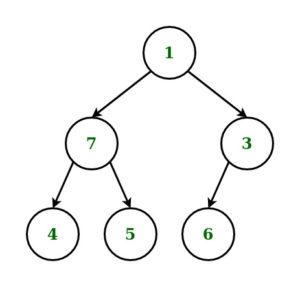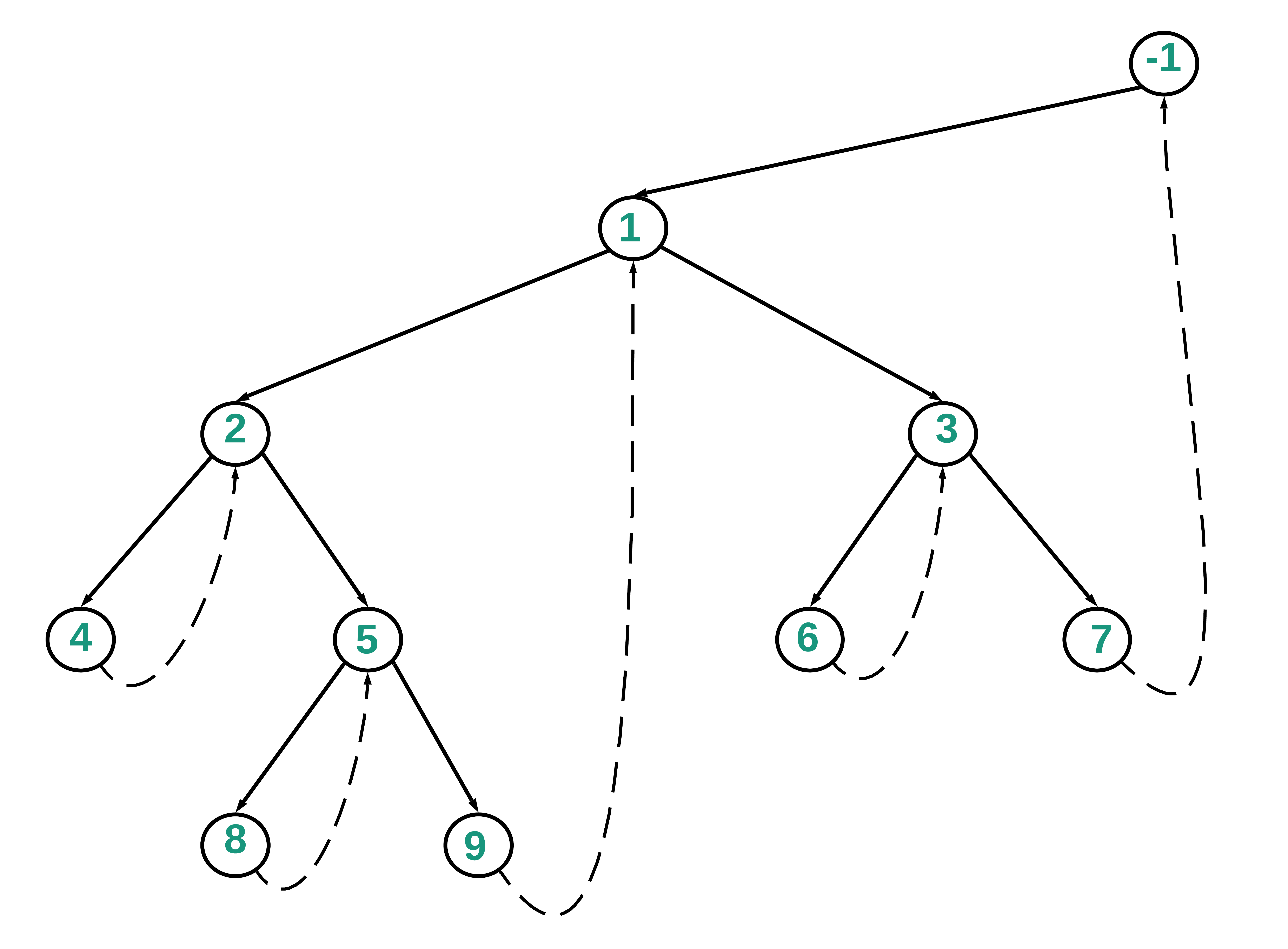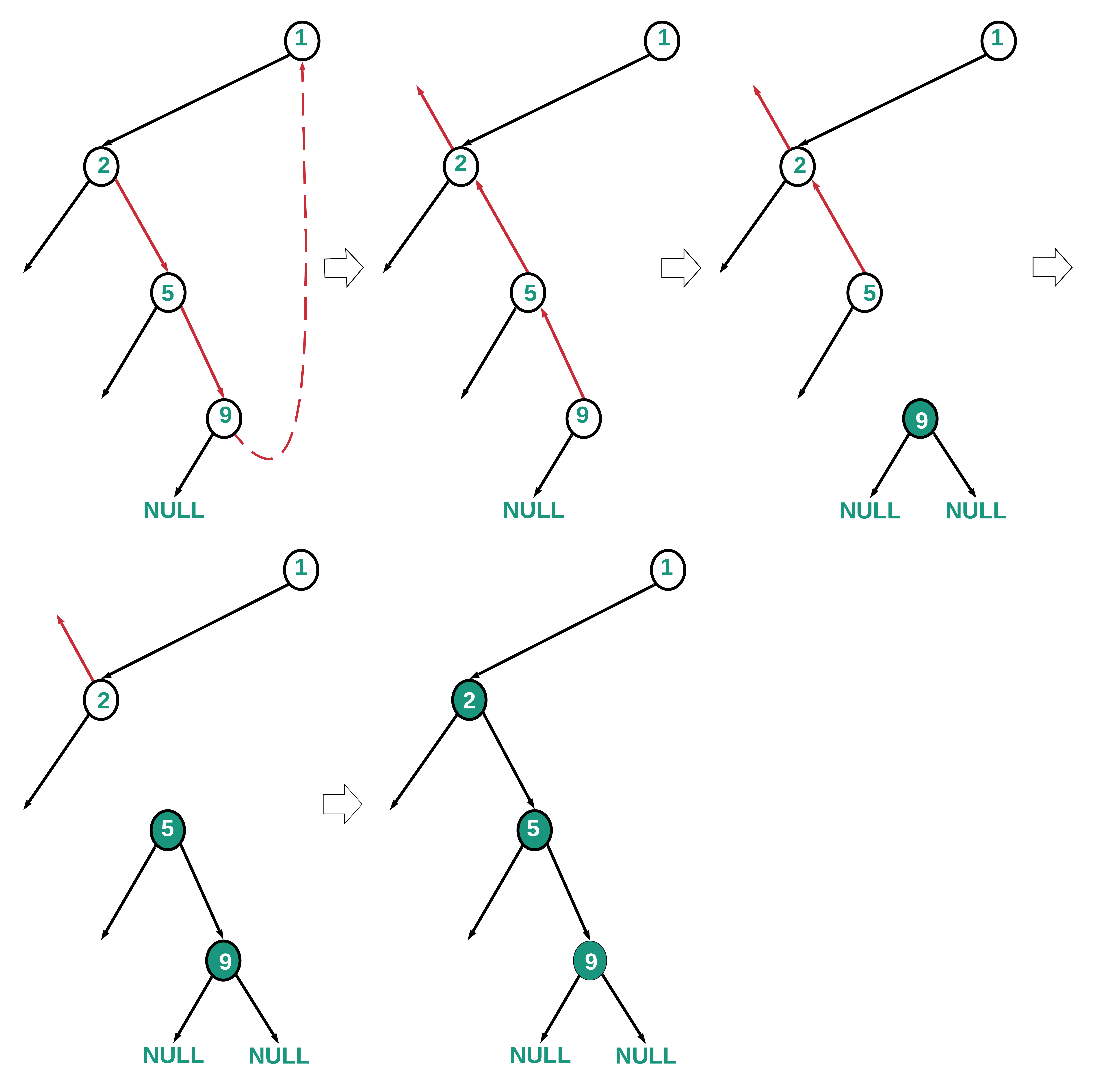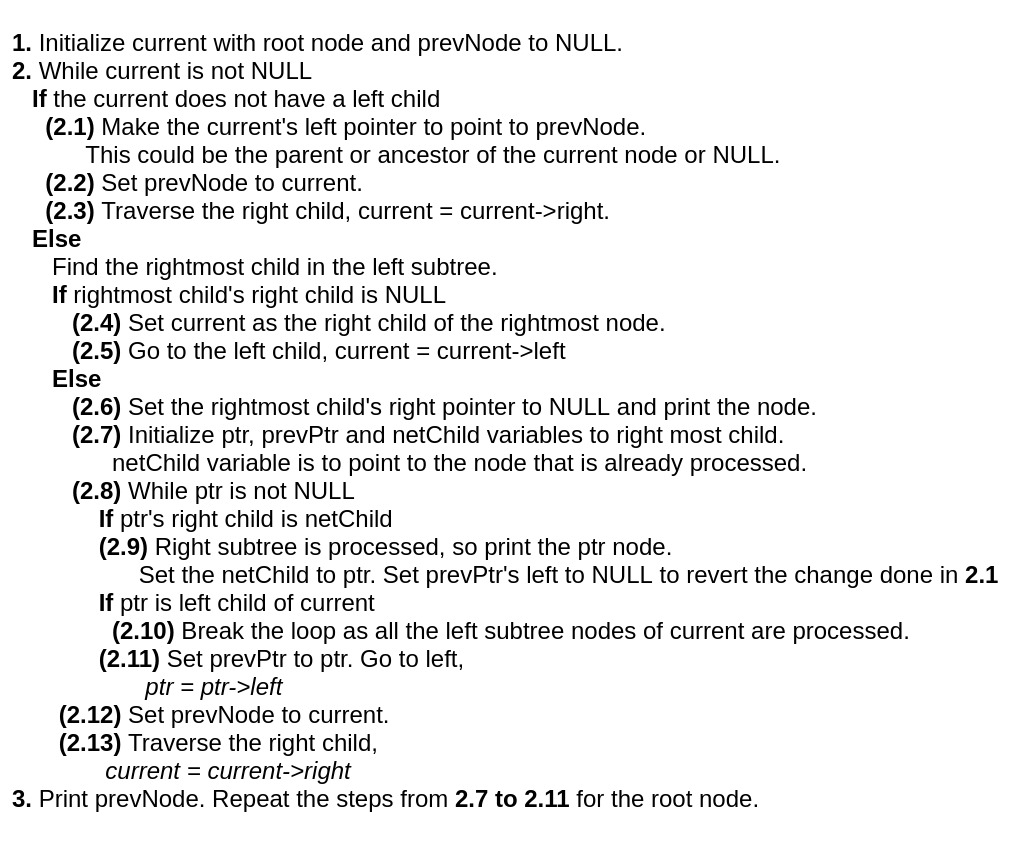1621840440

# Depth First & Tree Traversals (Pre, In, Post) Explained

In this video, we provide a walkthrough depth-first traversals of trees both iteratively and recursively and explore the differences across pre-order, in-order, and post-order traversals.

Highlights:

• 0:15 Learning Objectives
• 0:30 Depth-first traversal conceptual overview
• 5:30 Implementing depth-first traversal using a stack
• 9:58 Time and space complexity
• 10:50 Recursive implementation of depth-first traversal
• 15:31 Pre-order traversals
• 17:30 Post-order traversals
• 18:24 In-order traversals
• 20:12 Problem: summing a tree
• 28:14 Recap

#developer

## Buddha Community1598364000

## Mix Order Traversal of a Binary Tree

Given a Binary Tree consisting of** N** nodes, the task is to print its Mix Order Traversal.

Mix Order Traversal_ is a tree traversal technique, which involves any two of the existing traversal techniques like Inorder, Preorder and Postorder Traversal. Any two of them can be performed or alternate levels of given tree and a mix traversal can be obtained._

Examples:

_Input: _N = 6_Output: __7 4 5 1 3 6 _

Explanation:

_Inorder-Preorder Mix Traversal is applied to the given tree in the following order: _

_Inorder Traversal is applied at level 0 _

_Preorder Traversal is applied at level 1 _

Inorder Traversal at level 2._Output: __4 5 7 1 6 3 _

#data structures #recursion #tree #inorder traversal #postorder traversal #preorder traversal #tree traversals1600583760

## Tree Traversals in Kotlin

Below are the classic binary tree recursive traversal methods embedded into our BinaryNode class. We use a companion object to minimize our space complexity, which is comparable to static methods in Java. You may wonder why we allow for a nullable _BinaryNode _to be passed into each of these methods. I settled at this option for the sake of clarity. Because the left and right nodes are mutable objects, we would need to create immutable references to them before passing them to the recursive calls. You could say our base case is an empty leaf node.

The wonderful part about Kotlin is that we can define our action as a lambda expression. Below you will see an action defined as a parameter, which uses the value of the node being passed in as the first parameter. Our action here returns Unit, which is comparable to returning void in Java. Let’s look at how we can use this lambda expression.

``````class BinaryNode<T>(
var value: T,
var left: BinaryNode<T>? = null,
var right: BinaryNode<T>? = null
) {

companion object {

fun <T> traversePreorder(
node: BinaryNode<T>?,
action: (value: T) -> Unit
) {
if(node != null) {
action.invoke(node.value)
traversePreorder(node.left, action)
traversePreorder(node.right, action)
}
}

fun <T> traverseInorder(
node: BinaryNode<T>?,
action: (value: T) -> Unit
) {
if(node != null) {
traverseInorder(node.left, action)
action.invoke(node.value)
traverseInorder(node.right, action)
}
}

fun <T> traversePostOrder(
node: BinaryNode<T>?,
action: (value: T) -> Unit
) {
if (node != null) {
traversePostOrder(node.left, action)
traversePostOrder(node.right, action)
action.invoke(node.value)
}
}
}
}
``````

#preorder-traversal #n-ary-tree #kotlin #binary-tree #depth-first1595376000

## Post Order Traversal of Binary Tree in O(N) using O(1) space

Given a Binary Tree, the task is to print the elements in post order using O(N) time complexity and constant space.

``````Input:   1
/   \
2       3
/ \     / \
4   5   6   7
/ \
8   9
Output: 4 8 9 5 2 6 7 3 1

Input:   5
/   \
7       3
/ \     / \
4   11  13  9
/ \
8   4
Output: 4 8 4 11 7 13 9 3 5
``````

## Recommended: Please try your approach on {IDE} first, before moving on to the solution.

Method 1: Using Morris Inorder Traversal

1. Create a dummy node and make the root as it’s left child.
2. Initialize current with dummy node.
3. While current is not NULL
• If current does not have a left chil traverse the right child, current = current->right
• Otherwise,
1. Find the right most child in the left subtree.
2. If right most child’s right child is NULL
• Make current as the right child of the right most node.
• Traverse the left child, current = current->left
1. Otherwise,
• Set the right most child’s right pointer to NULL.
• From current’s left child, traverse along the right children until the right most child and reverse the pointers.
• Traverse back from right most child to current’s left child node by reversing the pointers and printing the elements.
1. Traverse the right child, current = current->right

Below is the diagram showing the right most child in left subtree, pointing to it’s inorder successor.Below is the diagram which highlights the path 1->2->5->9 and the way the nodes are processed and printed as per the above algorithm.Below is the implementation of the above approach:

• Java

filter_none

edit

play_arrow

brightness_4

`// Java program to implement`

`// Post Order traversal`

`// of Binary Tree in O(N)`

`// time and O(1) space`

`// Definition of the`

`// binary tree`

`**class**` `TreeNode {`

`**public**` `**int**` `data;`

`**public**` `TreeNode left;`

`**public**` `TreeNode right;`

`**public**` `TreeNode(``**int**` `data)`

`{`

`**this**``.data = data;`

`}`

`**public**` `String toString()`

`{`

`**return**` `data +` `" "``;`

`}`

`}`

`**public**` `**class**` `PostOrder {`

`TreeNode root;`

`// Function to find Post Order`

`// Traversal Using Constant space`

`**void**` `postOrderConstantspace(TreeNode`

`root)`

`{`

`**if**` `(root ==` `**null**``)`

`**return**``;`

`TreeNode current`

`=` `**new**` `TreeNode(-``1``),`

`pre =` `**null**``;`

`TreeNode prev =` `**null**``,`

`succ =` `**null**``,`

`temp =` `**null**``;`

`current.left = root;`

`**while**` `(current !=` `**null**``) {`

`// Go to the right child`

`// if current does not`

`// have a left child`

`**if**` `(current.left ==` `**null**``) {`

`current = current.right;`

`}`

`**else**` `{`

`// Traverse left child`

`pre = current.left;`

`// Find the right most child`

`// in the left subtree`

`**while**` `(pre.right !=` `**null**`

`&& pre.right != current)`

`pre = pre.right;`

`**if**` `(pre.right ==` `**null**``) {`

`// Make current as the right`

`// child of the right most node`

`pre.right = current;`

`// Traverse the left child`

`current = current.left;`

`}`

`**else**` `{`

`pre.right =` `**null**``;`

`succ = current;`

`current = current.left;`

`prev =` `**null**``;`

`// Traverse along the right`

`// subtree to the`

`// right-most child`

`**while**` `(current !=` `**null**``) {`

`temp = current.right;`

`current.right = prev;`

`prev = current;`

`current = temp;`

`}`

`// Traverse back from`

`// right most child to`

`// current's left child node`

`**while**` `(prev !=` `**null**``) {`

`System.out.print(prev);`

`temp = prev.right;`

`prev.right = current;`

`current = prev;`

`prev = temp;`

`}`

`current = succ;`

`current = current.right;`

`}`

`}`

`}`

`}`

`// Driver Code`

`**public**` `**static**` `**void**` `main(String[] args)`

`{`

`/* Constructed tree is as follows:-`

`1 `

`/     \`

`2       3`

`/ \     / \`

`4   5   6   7`

`/ \`

`8   9`

`*/`

`PostOrder tree =` `**new**` `PostOrder();`

`tree.root =` `**new**` `TreeNode(``1``);`

`tree.root.left =` `**new**` `TreeNode(``2``);`

`tree.root.right =` `**new**` `TreeNode(``3``);`

`tree.root.left.left =` `**new**` `TreeNode(``4``);`

`tree.root.left.right`

`=` `**new**` `TreeNode(``5``);`

`tree.root.right.left`

`=` `**new**` `TreeNode(``6``);`

`tree.root.right.right`

`=` `**new**` `TreeNode(``7``);`

`tree.root.left.right.left`

`=` `**new**` `TreeNode(``8``);`

`tree.root.left.right.right`

`=` `**new**` `TreeNode(``9``);`

`tree.postOrderConstantspace(`

`tree.root);`

`}`

`}`

Output:

``````4 8 9 5 2 6 7 3 1
``````

_Time Complexity: _O(N)

Auxiliary Space:_ O(1)_

Method 2: In method 1, we traverse a path, reverse references, print nodes as we restore the references by reversing them again. In method 2, instead of reversing paths and restoring the structure, we traverse to parent node from the current node using the current node’s left subtree. This could be faster depending on the tree structure, for example in a right-skewed tree.

The following algorithm and diagrams provide the details of the approach.#data structures #recursion #tree #inorder traversal #interview-preparation #morris-traversal #postorder traversal1599043260

## Check if all the Nodes in a Binary Tree having common values are at least D distance apart

Given a Binary Tree and an integer D, the task is to check if the distance between all pairs of same node values in the Tree is ? D or not. If found to be true, then print Yes. Otherwise, print No.

Examples:

Input:_ D = 7 _

``````                1
/   \
2     3
/ \   /  \
4   3  4   4

``````

Output:_ Yes _

Explanation:

_The repeated value of nodes are 3 and 4. _

_The distance between the two nodes valued 3, is 3. _

_The maximum distance between any pair of nodes valued 4 is 4. _

Therefore, none of the distances exceed 7

Input:_ D = 1 _

``````          3
/ \
3   3
\
3

``````

Output:_ No _

Recommended: Please try your approach on {IDE} first, before moving on to the solution.

**Approach: **

The idea is to observe that the problem is similar to finding the distance between two nodes of a tree. But there can be multiple pairs of nodes for which we have to find the distance. Follow the steps below:

1. Perform the Post Order Traversal of the given tree and find the distance between the repeated pairs of nodes.
2. Find the nodes that are repeated in the tree using unordered_map.
3. For each repeated node of a particular value, find the maximum possible distance between any pair.
4. If that distance is > D, print “No”.
5. If no such node value is found having a pair containing that value, exceeding **D, **then print “Yes”.

#greedy #recursion #searching #tree #binary tree #frequency-counting #postorder traversal #tree-traversal1598227740

## Construct a Maximum Binary Tree from two given Binary Trees

Given two Binary Trees, the task is to create a Maximum Binary Tree from the two given binary trees and print the Inorder Traversal of that tree.

What is the maximum Binary Tree?

_The __maximum binary __is constructed in the following manner: _

_In the case of both the Binary Trees having two corresponding nodes, the maximum of the two values is considered as the node value of the Maximum Binary Tree. _

_If any of the two nodes is NULL and if the other node is not null, insert that value on that node of the Maximum Binary Tree. _

Example:

``````Input:
Tree 1                Tree 2
3                    5
/ \                  / \
2   6                1   8
/                      \   \
20                      2   8
Output: 20 2 2 5 8 8
Explanation:
5
/ \
2   8
/ \   \
20   2   8

To construct the required Binary Tree,
Root Node value: Max(3, 5) = 5
Root->left value: Max(2, 1) = 2
Root->right value: Max(6, 8) = 8
Root->left->left value: 20
Root->left->right value: 2
Root->right->right value: 8

Input:
Tree 1            Tree 2
9                 5
/ \               / \
2   6             1   8
/ \                 \   \
20  3                 2   8
Output:  20 2 3 9 8 8
Explanation:
9
/ \
2   8
/ \   \
20  3   8

``````

#data structures #mathematical #recursion #tree #preorder traversal #tree traversals